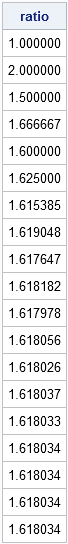This morning I read an interesting post about the design of the new Twitter Web page. The post included some R code to generate the ratio between adjacent terms in the Fibonacci seqence. The ratio converges to the "Golden Ratio": 1.61803399....

I'm sure that many R gurus will post simpler versions of the R code used in the post. In SAS, I would probably use the DATA step to generate the Fibonacci seqence and the ratio between adjacent terms, but since this is a blog about SAS/IML, here is some SAS/IML code:

```proc iml; n = 20; /* number of terms in the sequence */ fib = j(n,1); /* allocate; fill with 1's */ do i = 3 to n; fib[i] = sum(fib[i-1:i-2]); /* define sequence */ end; /* compute ratio of adjacent terms */ idx = 1:n-1; ratio = fib[idx+1] / fib[idx] ; print ratio[format=8.6];```Share